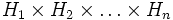# Order statistics-equivalence is finite-direct product-closed

## Statement

### Statement for direct product of two groups

Suppose$G_1, G_2, H_1, H_2$ are finite groups such that$G_1$ is order statistics-equivalent to$H_1$ and$G_2$ is order statistics-equivalent to$H_2$. Then, the External direct product (?)$G_1 \times G_2$ is order statistics-equivalent to the external direct product$H_1 \times H_2$.

Here, two finite groups are termed order statistics-equivalent finite groups if they have the same order statistics, i.e., the same number of elements of each order.

### Statement for direct product of finitely many groups

Suppose$G_1, G_2, \dots, G_n$ and$H_1, H_2, \dots, H_n$ are groups such that, for each$i$ satisfying$1 \le i \le n$,$G_i$ is order statistics-equivalent to$H_i$. Then, the External direct product (?)$G_1 \times G_2 \times \dots \times G_n$ is order statistics-equivalent to$H_1 \times H_2 \times \dots \times H_n$.